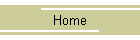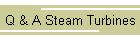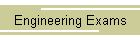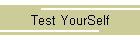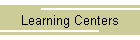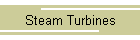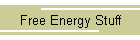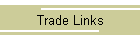WWW.MASSENGINEERS.COM2nd Class Engineer Written Test 02/19/2002  1.)   Conduct an over speed trip on a turbine. 2.)   How do you calculate water rate? 3.)   You have 52 barrels of fuel oil with a specific gravity of .85. What does the fuel weigh? (1 gallon of water weighs 8.33 lbs). 4.)   What does ANSI 31.1 say about blow off valves and piping on a boiler with an M.A.W.P. of 125 PSI? 5.)   What is the factor of safety of a boiler with an M.A.W.P. of 275 PSI that has a safety valve set at 150 PSI? The old factor of safety was 4. 6.)   When a process goes through volume and pressure variations but no temperature changes is called? 7.)   Describe the construction and operation of a Kingsbury Thrust Bearing. 8.)   Which turbine has more end thrust Impulse or Reaction and why?   9.)   What is “Water Hammer” and how do you prevent it and remedy it? 10.)  A direct stay 1” in diameter is attached to a flat surface of an HRT boiler. Would the diameter of a diagonal stay be supporting the same area? The diagonal stay is 60” in length and the length of the line drawn at a right angle to the surface supported is 48”  (Answer is PFT – 32 ASME Code Section one) 11.) What is the horsepower of a 1000 Kilowatt Generator? 12.) When a process goes through Volume and Pressure variations but no temperature change occurs this is known as? (Yes the question was repeated on the second page of the test). 13.) How do we calculate Turbine horsepower and what is water rate used for? (Yes, second water rate question). 14.) What is the first law of Thermodynamics? 15.) Calculate the M.A.W.P. for a boiler 60” in diameter, having ½” thick plate, with a tensile stress of 11,000 and a joint efficiency of 80%? Use p=.8SET/R + 6T 16.) Explain Factor of Evaporation and what is it used for? 17.) Define the following; Tensile strength, Elastic limit, Yield point, Elongation and Reduction of area? 18.)  A.) What is minimum relieving capacity of an electric boiler operating at 512 Watts?Copy & Paste the question above into the search box to find your answer.   Disclaimer Remember it is how the applicants remembered the questions. Note: Exams are not the original content may be different.  Visit www.massengineers.com for more engineering exams and job opportunities.Massengineers website now has out of print, and used engineering books sell.  Hard to find classic and collectible steam engineering, power plant, steam boiler books.  Send Exams to RussReeves@massengineers.com